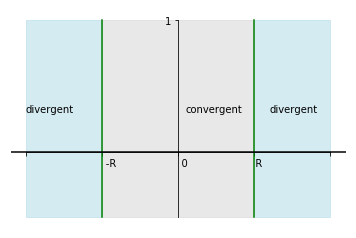Notebook

# Maths

## Series

The following chapter shows various series and how to handle them. The code used can be found here.

In :
% run ./maths_functions.py


### Arithmeic Series

Members are:

$a_n = a_{n-1}+d$

Sum is:

$S_n = (a_n+a_1).n/2$

### Geometric Series

$a_n = a_{n-1}.q$

$S_n = a_1.\cfrac{(q^n-1)}{(q-1)}$

$S_{\infty} = \cfrac{a}{1-r}$

### Power Series

Power series is an infinite series of the type:

$a_0 + a_1.(x-c) + a_2.(x-c)^2 + \dots + a_n.(x-c)^n$, where the coeffcients $a_n$ are independent of $x$ and $c$ is a constant.

The general formulation is often seen as Taylor Series of an unknown function, while in the case when $c=0$, this series is also called a Maclaurin Series.

Radius of convergence is the largest power of x within a Power Series with which the series converges.

if |$x$| < $R$ -> convergent

if |$x$| > $R$ -> divergent

if |$x_1$| > |$x_0$| -> convergent

if |$x_1$| < |$x_0$| -> divergent

In :
convergence_example()#### Integration of a Power Series

Power series: $S_n = \sum_0^n a_n.x^n$

Integral: $\int S_n dx = S_m = \sum_0^n b_n.x^n$, where $b_n = (a_n-1)/n$

### Taylor Series Approximation

For any given function $f(x)$, an approximation $P_n(x)$ exists that is a sum of an infinite number of terms of the derivatives of the function at a given point $a$.

$P_n = f(a) + (x-a).f'(a) + (x-a)^2.f''(a)/2 + ...$

$P_n = \sum_0^n \frac{(f^{(n)}(a).(x-a)^n)}{n!}$

For $x=0$, this function is also MacLaurin Series.

For two variables, the Taylor Series expands like this:

$P_n = f(a,b) + h.f_x(a,b) + k.f_y(a,b) + f_{xx}(a,b).h^2/2 + f_{yy}(a,b).k^2/2 + f_{xy}(a,b).h.k + ...$

#### Analysis

To solve, find roots of $f_x=0$ and $f_y=0$.

Substitute into $D=f_{xx}.f_{yy}-f_{xy}.f_{yx}$

if D < 0, it's a saddle point,

if D = 0, it is inconclusive,

if D > 0 and $f_{xx}$ > 0, it's a minimum,

if D > 0 and $f_{xx}$ < 0, it's a maximum.